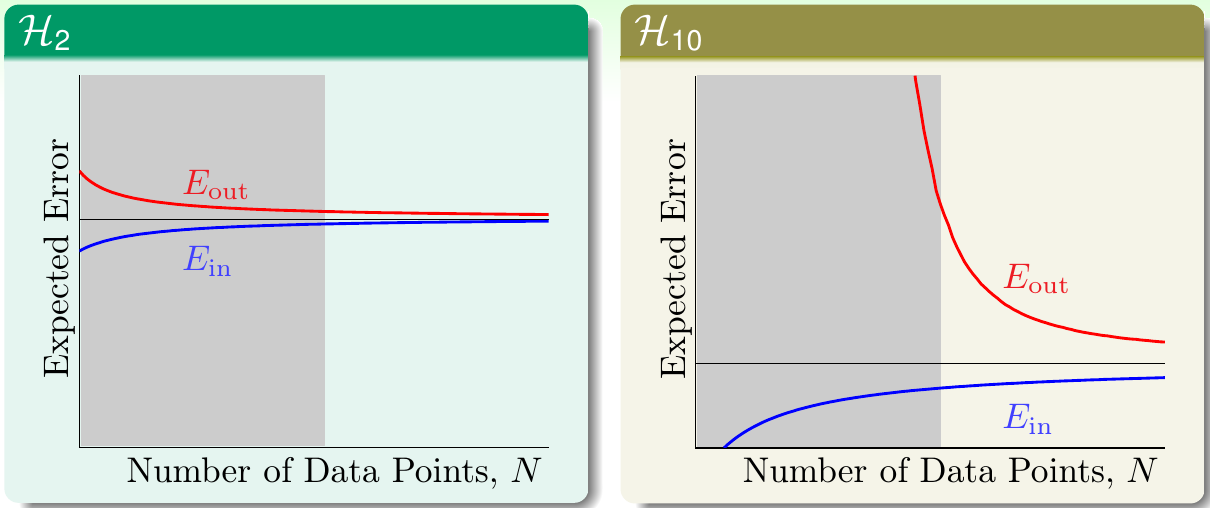## 什么是过拟合

• 过拟合，类似于出车祸
• 使用太复杂，$d_{\rm VC}$太大的模型，类似于开太快
• 数据中噪声比较多，类似于路面情况不平
• 数据集大小$N$比较小，类似于司机对路面状况观察比较有限，对路况不熟

## 噪声与数据集大小的角色

• 目标函数是某个十次多项式，数据产生时加入一些噪声，产生15个数据点
• 目标函数是某个50次多项式，数据产生时不加入噪声，产生15个数据点• 随着数据量$N$的增长，$E_{\rm out}$会下降，$E_{\rm in}$会上升。最后当$N\rightarrow \infty$时，$|E_{\rm in} - E_{\rm out}| \rightarrow 0$
• $E_{\rm in}$永远小于$E_{\rm out}$。因为模型在学习数据时，总会学习到其所看见的数据中的噪声，并对其进行拟合。而对看不见的数据就难以对噪声拟合很好。由于假设数据集噪声的期望为0，因此当偏向一面时，就会远离另一面
• $\mathcal{H}_{10}$$E_{\rm in}$永远低于$\mathcal{H}_2$

## 确定性噪声

• 数据量太小
• 随机噪声太大
• 确定性噪声太大
• 使用的模型比目标函数更复杂（注意第二幅图左下角）

## 应对过拟合

• 先使用简单模型训练，类似于开慢车
• 对数据做一些清洗，类似于使用更准确的路况
• 观察数据，根据对数据的理解，按照相同规律产生一些新的数据（data hinting），类似于多看一些路况
• 使用正则化方法，类似于踩刹车
• 验证模型，类似于常看仪表盘